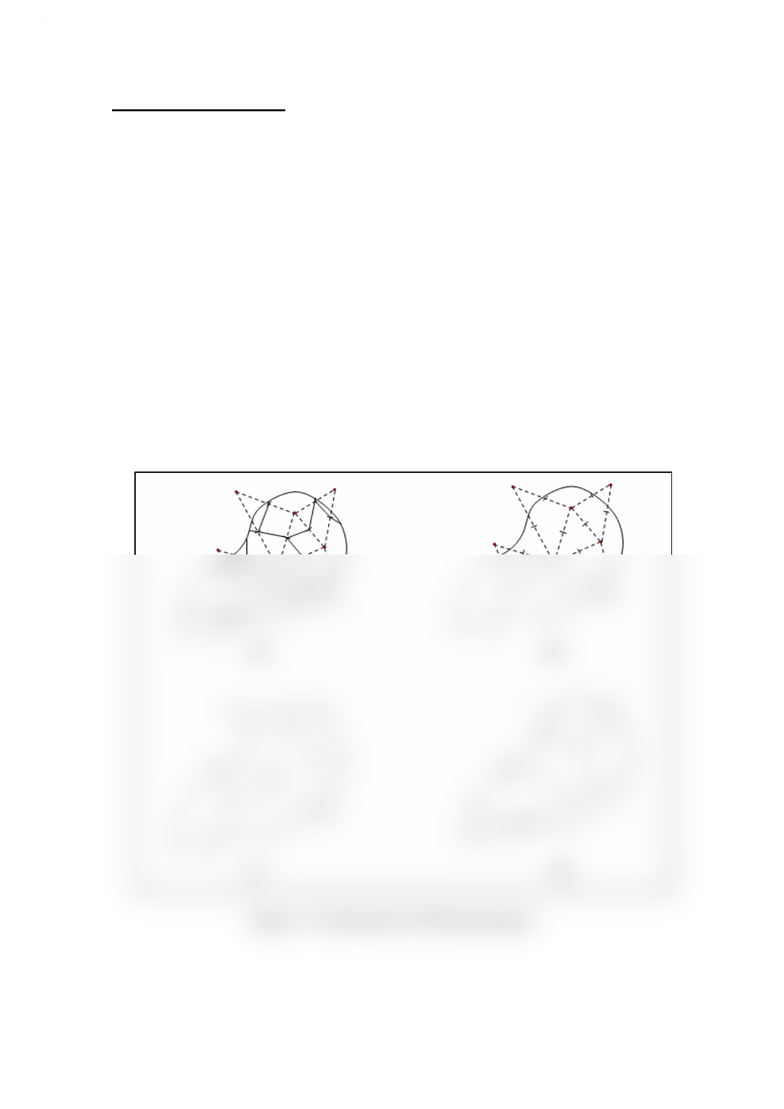Textbook Notes (290,000)
CA (170,000)
UTSG (10,000)
Geography (100)
Chapter

# GGR252H1 Chapter Notes -Voronoi Diagram

Department
Geography
Course Code
GGR252H1
Professor
Stephen Swales

This preview shows half of the first page. to view the full 2 pages of the document.Thiessen polygon Method
In order to achieve accurate estimation of the spatial distribution of rainfall, it is
necessary to use interpolation methods, for this, the Thiessen* method is considered as
the most important in engineering praxis.
This method assigns weight at each gauge station in proportion to the catchment area
that is closest to that gauge.
The method of constructing the polygons implies the following steps:
1. Gauge network is plotted on map of the catchment area of interest.
2. Adjacent stations are connected with lines.
3. Perpendicular bisectors of each line are constructed (perpendicular line at the
midpoint of each line connecting two stations)
4. The bisectors are extended and used to form the polygon around each gauge station.
5. Rainfall value for each gauge station is multiplied by the area of each polygon.
6. All values from step 5 are summed and divided by total basin area.
An example of spatial precipitation distribution according to Thiessen method can be
appreciated in the following figure.
Figure 2.3 Construction of Thiessen polygon